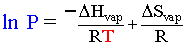Enthalpy and Entropy of Vaporization of Water

The purpose of this experiment is to determine the enthalpy and entropy of vaporization of water.

Entropy ( ΔSvap ) is a concept we have not yet covered in class. For the purposes of this lab, read section 19.2 in your text. Think of it as just another value for vaporization, similar but different that enthalpy of vaporization, ΔHvap.

Liquid vapor pressure comes about from molecules near the surface of the liquid having enough kinetic energy to liberate themselves from the iron grip of intermolecular forces in the liquid. As the temperature of the liquid increases, the vapor pressure also increases as more molecules have the minimum amount of energy to be liberated. The vapor pressure of a liquid at any temperature is also mathematically correlated with the enthalpy ΔHvap and entropy ΔSvap of  vaporization. The Clausius-Clapeyron equation describes this relationship:Everything in black is a constant in the above equation. Therefore if we make a graph of 1/T (x) vs. ln P (y) a straight line should exist with the slope correlated to ΔHvap and the y-intercept correlated to ΔSvap The R you must use in this case is 8.314 J/K mol. Remember that who kJ, J conversion thing.

The procedure will involve the measuring of a sample of gas (air + water vapor) at various temperatures. You will place an sealed flask with a small amount of water in it into a container of hot water and connect a pressure sensor to it.  Water will evaporate until it the vapor pressure of water (at that high T) is reached. You will then let it cool. As the temperature decreases, the vapor pressure of water will decrease as water condenses. Our pressure sensor will iondicate a decrease in TOTAL pressure, however, the pressure of the air that was already in the flask will also be decreasing which is going to be an issue. This means that we will have to calculate the PH2O at each step, as the pressure sensor measures total pressure at that temperature (Ptotal = Pair + PH2Ovapor

Here is an 8 min screencast of the experimental 'procedure'

The calculations in this experiment are ugly, and doing them by hand is a pain. Your instructor will explain in lab how to do the data manipluation, but for now know that the values you will need to calculate (in your spreadsheet) for each data point are:

- Absolute temperature
- Partial pressure of air sample (knowing nair, T and V and using PV=nRT)
- Partial pressure of water vapor (Ptotal - Pair)
- Inverse absolute temperature (1/T) (for graph)
- ln PH2O (for graph)

If you do it in columns, you can use these last two columns to get your straight line graph.

Lab report: I'm feeling nice, so you need to give me a summary, results and commentary sections only. I want % error for each value for each 'good' run you did, so no average or SD are needed. The ΔHvap at this temperature (65-70C) is actually 42.0 kJ/mol. That is the 'known' value. ΔSvap is not temperature dependant so you can easily look that up.You need not give actual raw data nor sample calculations.

Prelab questions: (to be completed on a separate piece of recycled paper, handed to the instructor as you enter lab)

0. You will need to bring a portable memory device (flash drive for example) to lab to extract teh data from the equipment so you can mnaipulate it elsewhere.
1. Ethanol has a
ΔHvap 38.56 kJ/mol and ΔSvap = 109.67 J/K mol. What is the vapor pressure of ethanol at 300. K? Show all your work. Watch your units! The units on the right hand side of the Clausius-Clapeyron equation all cancel out but is still equal to Ln P (which you would think needs a unit) That problem comes from the fact that you cannot take a logarithm of a unit. When you solve for the right hand side and take the anti-log, the units are atm. Thats is a product of the way the equation is derived.
2. Write, in your own words, the 'procedure' as explained in the screencast.

~MEO 23Jan08